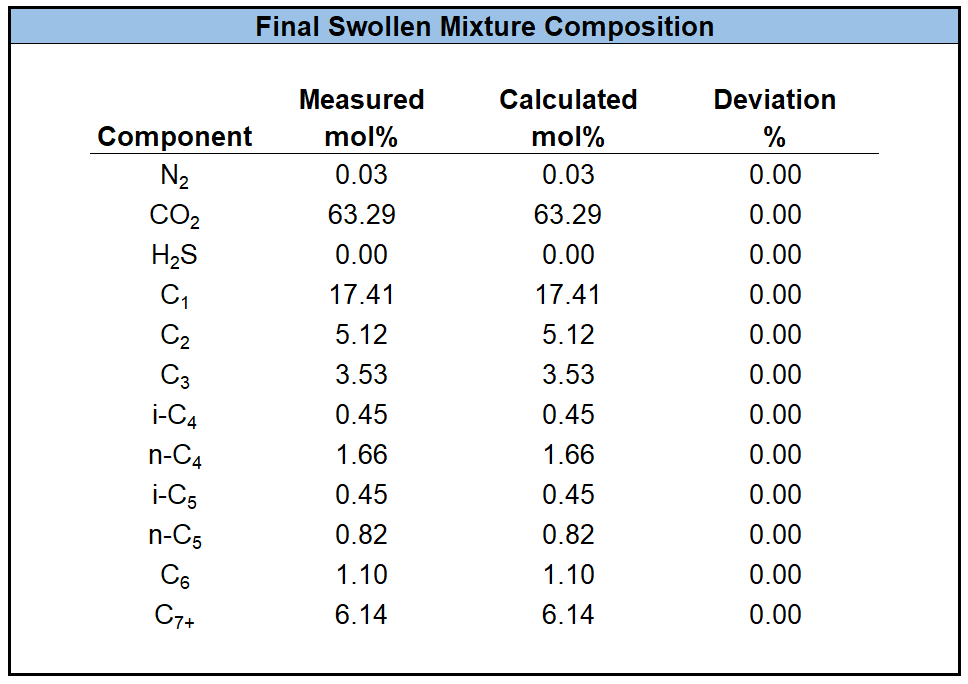# Swell Test Experiment

In the context of miscibility, a swell test can be used to estimate the first contact minimum miscibility pressure (MMP$$_{FC}$$). The general concept of a swell test is to take an initial reservoir fluid and mix an injection fluid (typically a relevant injection gas) to estimate the miscibility effects of the given injection fluid with the reservoir fluid. Normally the results are given in a swell test plot (see Figure 1). A swell test plot shows the saturation pressure and type with respect to the relative moles injected (RMI). The definition of the RMI is given by

where $$F_{inj}$$ is the fraction of injected gas with respect to the entire number of moles in the mixture (i.e. $$F_{inj}=n_{inj}/(n_{inj}+n_{RF})$$).

## Procedure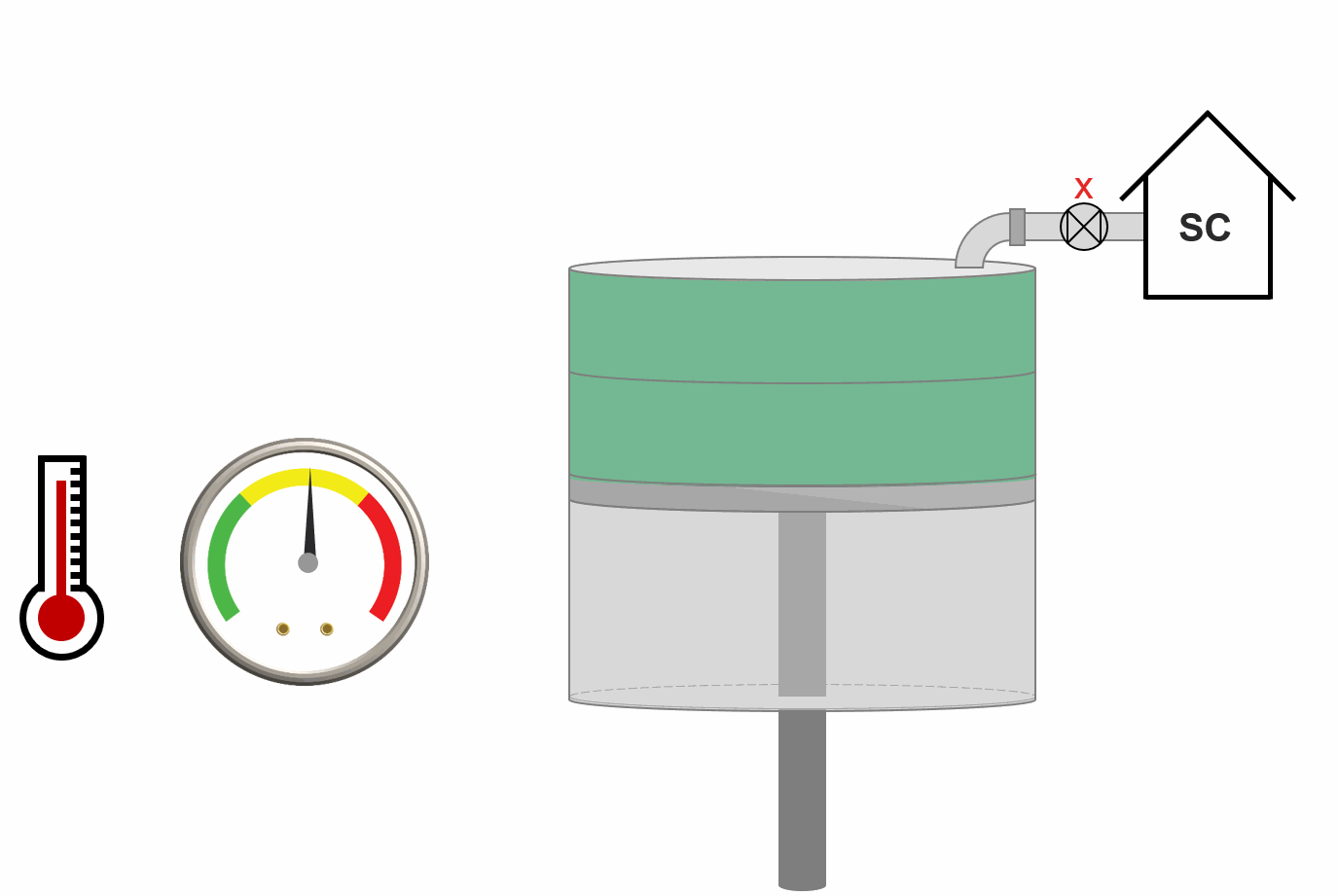Animation 1: Animation of swell test procedure.

The procedure of the swell test is given as a step-by-step guide below and is shown in Animation 1 for a single step in the procedure.

1. Initiate reservoir fluid at pressure and temperature.

2. Mix fluid in step 1 with increment of injection gas.

3. Pressurize mixture in step 2 to some pressure above the saturation pressure.

4. Decrease the pressure by applying a CCE to estimate the saturation pressure and saturation type.

5. Record saturation pressure estimate and saturation type with the relative moles injected and add to the swell test plot (see Figure 1).

6. Take the new mixture at its saturation pressure and return to step 2.

## Results

Once the swell test data is found, the MMP by first contact is determined by the largest value of the swell test data. An example of the swell test data and the MMP by first contact is given in Figure 1.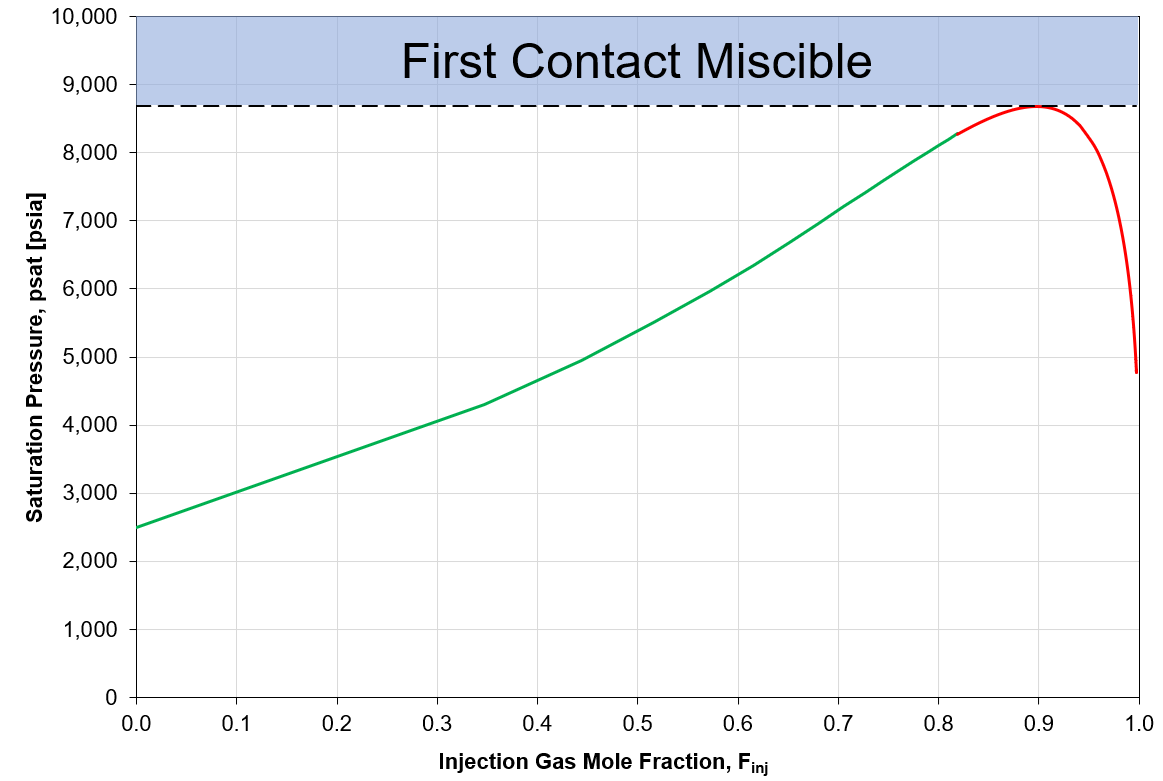Figure 1: Example of swell test data and indicated MMP by first contact.

Note that there is some experimental evidence showing asymptotic behavior of the swell test curve (i.e. the curve "blows up") which yields no MMP$$_{FC}$$.

## Quality Control / Quality Assurance

To check the consistency of the data, the mixture density and the final swollen mixture composition is back-calculated using material balance. Then, the results are compare against the reported values.

Let's do these step by step. Table 1 shows a data template for a swell test.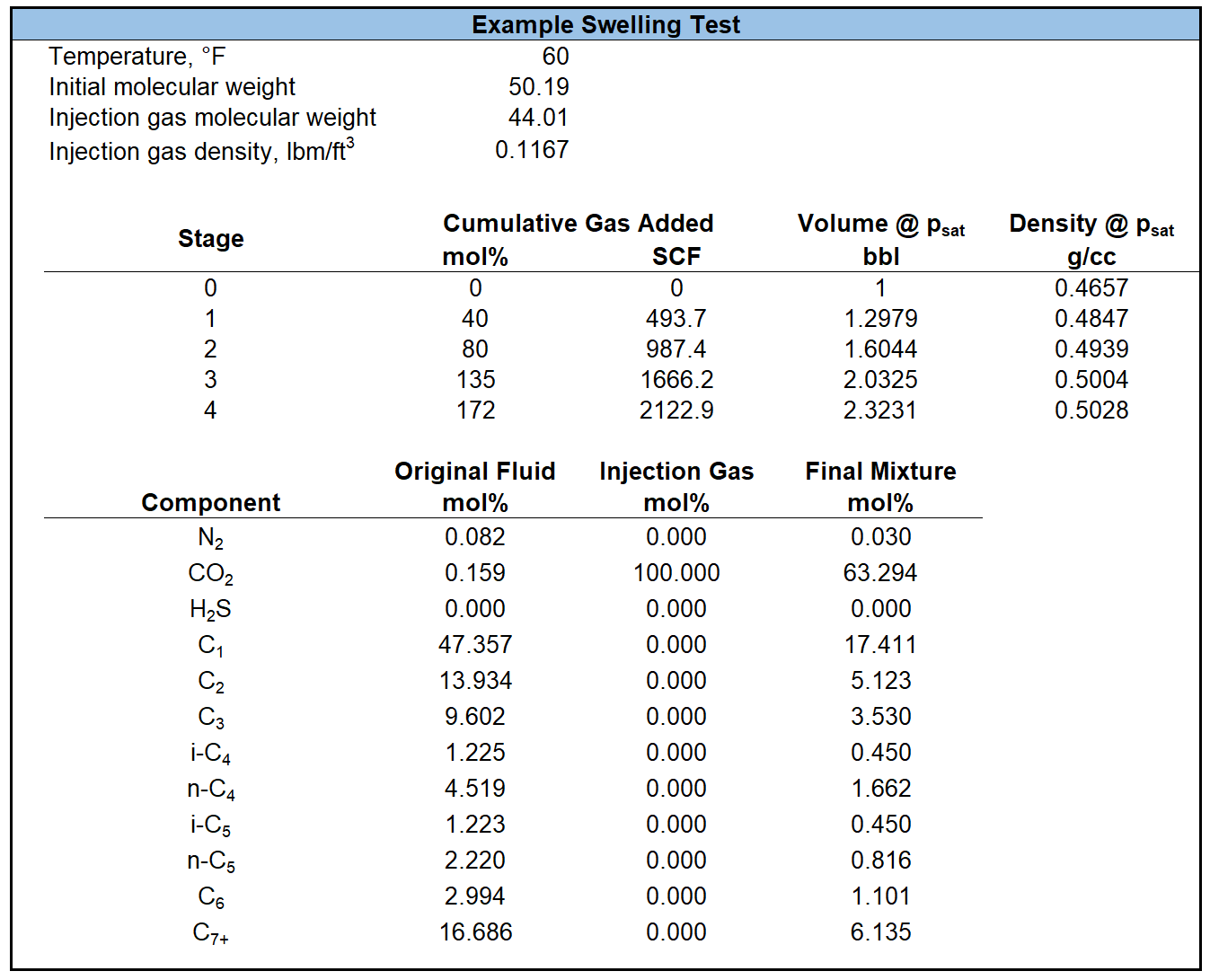### Mixture Density

To check the quality of the reported mixture density, it is back-calculated using material balance. To calculate the mixture density, cumulative mass of the injected gas is divided by initial volume.

Initial mass in the cell is given by the definition of the density.

The mass is converted to the moles using the initial molecular weight.

Cumulative injected mass is calculated using the cumulative injected volume and the density of the injection fluid as

If $$\rho_{\text{inj}}$$ is not given, it is estimated by the ideal gas law.

If $$V_{\text{cum,inj}}$$ is not given, the injected mass is calculated using the cumulative mole ratio $$F_{\text{inj}}$$.

Now, the mixture density is the mixture mass (initial mass + injected mass) divided by the volume.

Usually, the mixture density is plotted against the injection gas mole fraction $$f_{\text{inj}}$$ that is given by

If the added gas mole ratio $$F_{\text{inj}}$$ is not reported, it is calculated by

where, the injected moles is given by

### Final Mixture Composition:

To check the quality of the reported final mixture composition, it is back-calculated using material balance equation. To calculate the final mixture composition, the initial oil and the injected gas compositions are mixed using the mole fraction of the injected gas at the final stage.

The molar composition of the component $$i$$ in the liquid mixture at the final stage is given by

where, $(x_{i})_{\text{initial}}$ is the molar composition of the component $i$ in the initial liquid, $(y_i)_{\text{inj}}$ is the molar composition of the component $$i$$ in the injection fluid. $(f_{\text{inj}})_{\text{final}}$ is injection gas mole fraction given by Equation \eqref{eq:injection_gas_mole_fraction}.

By implementation of the procedure given above, the following results will will be obtained.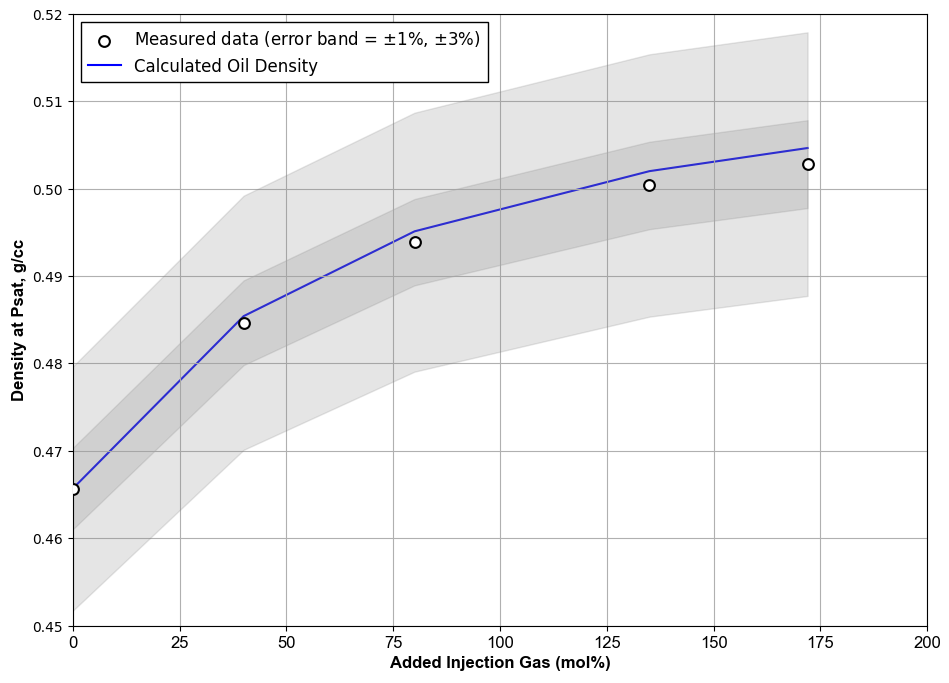Figure 1: Example of swell test density QC.

Table 2: Material balance QC for final swollen mixture composition